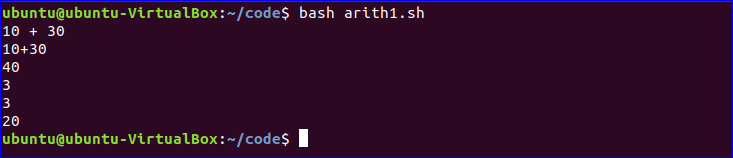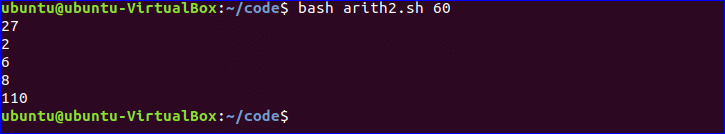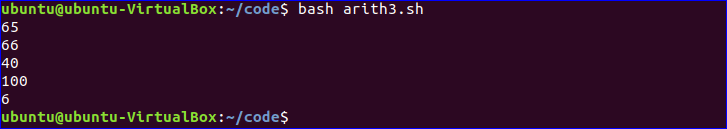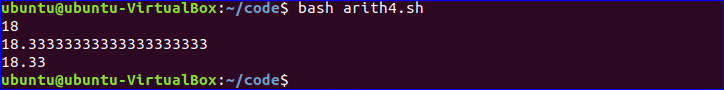# Bash Arithmetic Operations

Doing arithmetic operations in bash is not similar to other standard programming languages. One of the limitations of bash is that it can’t handle floating point or double numbers like other scripting languages. Another command tool is used in bash to solve this problem. Different types of arithmetic operations are shown in this tutorial by using different examples.

### Example – 1: Using ‘expr’ command

The oldest command for doing arithmetic operations in bash is ‘expr’. This command can work with integer values only and prints the output directly in the terminal. You have to use space with each operand when you want to use ‘expr’ command to do any mathematical operations. Create a bash file and add the various ‘expr’ commands to check how the ‘expr’ command works.

#!/bin/bash

# Works as string
expr '10 + 30'

# Works as string
expr 10+30

#Perform the addition
expr 10 + 30

#Find out the remainder value
expr 30 % 9

#Using expr with backtick
myVal1=`expr 30 / 10`
echo \$myVal1

#Using expr within command substitute
myVal2=\$( expr 30 - 10 )
echo \$myVal2

Run the file arith1.sh.

\$ bash arith1.sh

Output:

The output shows that arithmetic operators worked only when space is used with each numeric value and no single quotation is used with expr command. You can also assign the output of expr command into a variable and print the variable later by using backtick or command substitute. 30/10 is calculated by using backtick and 30-10 is calculated by using command substitute.### Example – 2: Using ‘let’ command

‘let’ is another built-in command to do arithmetic operations in bash. ‘let’ command can’t print the output to the terminal without storing the value in a variable. But ‘let’ command can be used to remove the other limitations of the ‘expr’ command. Create a bash file and add the following code for seeing how the ‘let’ command works.

#!/bin/bash

# Multiplying 9 by 8
let val1=9*3
echo \$val1

# Dividing 8 by 3
let "val2 = 8 / 3"
echo \$val2

# Subtracting 3 from 9
let val3=9-3
echo \$val3

# Applying increment
let val4=7
let val4++
echo \$val4

# Using argument value in arithmetic operation
let "val5=50+\$1"
echo \$val5

Run the file arith2.sh.

\$ bash arith2.sh

Output:

The output shows that ‘let’ command is more flexible than the ‘expr’ command. You can evaluate any arithmetic expression with or without quotations. But you can’t use space within any mathematical expression. You can use the increment or decrement operator in ‘let’ command. How the arithmetic operation can be done with argument values using ‘let’ command is shown in the last part of the example.### Example – 3: Using double brackets

You can perform any arithmetic operation in bash without using any command. Here, double brackets are used to do the arithmetic tasks and using double bracket for executing mathematical expressions is more flexible than commands like ‘expr’ or ‘let’. Create a bash file and add the following code to test the arithmetic operations by using double brackets.

#!/bin/bash

# Calculate the mathematical expression
val1=\$((10*5+15))
echo \$val1

# Using post or pre increment/decrement operator
((val1++))
echo \$val1
val2=41
((--val2))
echo \$val2

# Using shorthand operator
(( val2 += 60 ))
echo \$val2

# Dividing 40 by 6
(( val3 = 40/6 ))
echo \$val3

Run the file arith3.sh.

\$ bash arith3.sh

Output:

The output shows that double brackets can execute any mathematical expression with space or without space and you can also use increment/decrement and shorthand operators in double brackets expressions.### Example – 4: Using ‘bc’ command for float or double numbers

One of the major limitations of the above ways of doing arithmetic operations in bash is that ‘expr’ or ‘let’ or double brackets expression are not able to produce floating point or double numbers. The output of division operations of the above examples are integers. ‘bc’ command can be used to solve this problem and it works as a basic calculator for the Linux operating system. Create a bash file and add the following code to check the use of ‘bc’ command in arithmetic operations.

#!/bin/bash

# Dividing 55 by 3 with bc only
echo "55/3" | bc

# Dividing 55 by 3 with bc and -l option
echo "55/3" | bc -l

# Dividing 55 by 3 with bc and scale value
echo "scale=2; 55/3" | bc

Run the file arith3.sh.

\$ bash arith4.sh

Output:

The output shows that simple ‘bc’ command produces integer value like other options when any division expression is executed. ‘bc -l’ command generates exact output of the division and you can limit the fractional part by using scale value. Here, scale=2 is used. So the output shows 2 digits after decimal point.You can apply any of the mentioned ways to perform arithmetic operation in bash based on your requirements.

### About the author#### Fahmida Yesmin

I am a trainer of web programming courses. I like to write article or tutorial on various IT topics. I have a YouTube channel where many types of tutorials based on Ubuntu, Windows, Word, Excel, WordPress, Magento, Laravel etc. are published: Tutorials4u Help.